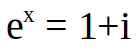# Math Contest #21 Results and Solution

in puzzle •  9 months ago

## Solution

The problem of this contest was to find the logarithm of a complex number:This can be done easily using euler's formula:
`e^(x+iy) = e^x * (cos(y) + i*sin(y))`
`= e^x *cos(y) + e^x *i*sin(y) = a+bi`
`tan(y) = sin(y)/cos(y) = b/a``y = tan⁻¹(b/a)` ,
And becuase cos²(x)+sin²(x) = 1 :`e^x = |a+bi| = sqrt(a²+b²)`
`x = log(sqrt(a²+b²))`

Now entering the given values into the function leads to:
`x = log(sqrt(1²+1²)) = log(sqrt(2))`,
`y = tan⁻¹(1/1) = tan⁻¹(1) = 45° = π/4`
Of course you can now also plug the log(sqrt(2)) in your calculator, but that's not necessary. log(sqrt(2)) is also infinitely more precise than anything your calculator could ever give you. And besides this contest should be possible to do without a calculator.

Because of the cyclical nature of tan(x) the result can also be shifted by any 2nπ ∀n ∊ ℤ:
y = π/4 + 2nπ

↓↑↓↑↓↑↓↑↓↑↓↑↓↑↓↑↓↑↓↑↓↑↓↑↓↑↓↑↓↑↓↑↓↑↓↑↓↑↓↑↓↑↓↑↓↑↓↑↓

### List of participants with their entries:

Namesolutions foundcomment
@golddeckresult from googleRejected, because you just plugged the log(1+i) into google and that is not the kind of problem solving that I want to encourage in this contest.
@kaeserotorcorrect

↓↑↓↑↓↑↓↑↓↑↓↑↓↑↓↑↓↑↓↑↓↑↓↑↓↑↓↑↓↑↓↑↓↑↓↑↓↑↓↑↓↑↓↑↓↑↓↑↓

## Winner draw:

Not necessary due to only one accepted entry:
Congratulations @kaeserotor , you won 3 SBI!
↓↑↓↑↓↑↓↑↓↑↓↑↓↑↓↑↓↑↓↑↓↑↓↑↓↑↓↑↓↑↓↑↓↑↓↑↓↑↓↑↓↑↓↑↓↑↓↑↓

### The next contest starts in 2 days. Don't miss it!

Sort Order:
·  9 months ago

Good one btw, whenever I solve one of your riddles, some old knowledge is refreshed. Really enjoying it!

Posted using Partiko Android

·  9 months ago

!sbi

Posted using Partiko Android